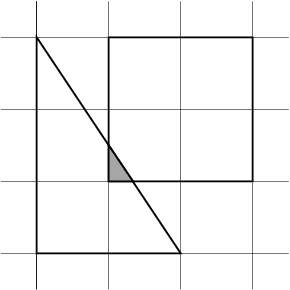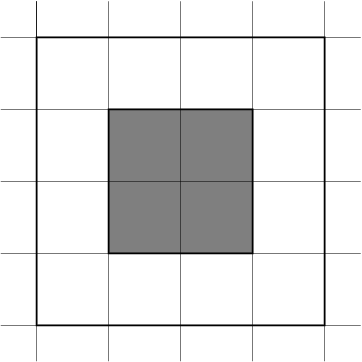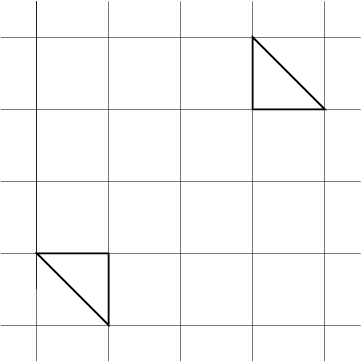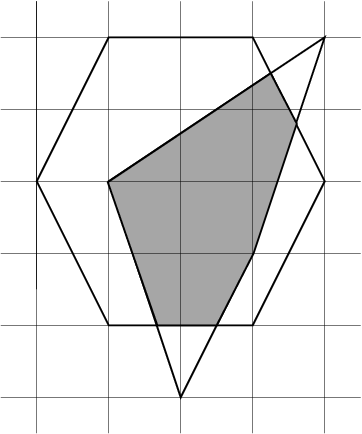### Problem Statement

You are given two polygons, described by two String[]s polygon1 and polygon2. The elements of polygonx (x=1 or 2) consist of two space separated integers describing the coordinates of the vertices of polygon x in counterclockwise order.

Both polygons will be convex and have non-zero areas. Furthermore the two polygons will be such that a vertex of one polygon won't lie on the sides of the other.

Return the area of the overlap of the two polygons.

### Definition

 Class: ConvexPolygons Method: overlap Parameters: String[], String[] Returns: double Method signature: double overlap(String[] polygon1, String[] polygon2) (be sure your method is public)

### Notes

-A polygon being convex means: every straight line joining any two interior points of the polygon is entirely contained in the interior of the polygon.
-A return value with either an absolute or relative error of less than 1e-9 is considered correct.

### Constraints

-polygon1 and polygon2 each have between 3 and 50 elements, inclusive.
-Each element of polygon1 and polygon2 has length between 1 and 50, inclusive.
-Each element of polygon1 and polygon2 consists of two space separated integers with values between -1000 and 1000, inclusive.
-Both polygons will be convex and have non-zero areas.
-Both polygons will be such that a vertex of one polygon won't lie on the sides of the other.
-The points described by polygon1 and polygon2 will be in counterclockwise order.

### Examples

0)

 `{"00 00","02 00","00 03"}` `{"1 1","3 1","3 3", "1 3"}`
`Returns: 0.08333333333333326`1)

 `{"-1 -1","1 -1","1 1","-1 1"}` `{"-2 -2","0 -2","2 -2","2 0","2 2","0 2","-2 2","-2 0"}`
`Returns: 4.0`
 When one polygon encloses the other, the overlap is the area of the smaller one.2)

 `{"-1 -1","-2 -1","-1 -2"}` `{"1 1","2 1","1 2"}`
`Returns: 0.0`
 They don't overlap.3)

 `{"-2 0","-1 -2","1 -2","2 0","1 2","-1 2"}` `{"0 -3","1 -1","2 2","-1 0"}`
`Returns: 5.233333333333333`#### Problem url:

http://www.topcoder.com/stat?c=problem_statement&pm=4559

#### Problem stats url:

http://www.topcoder.com/tc?module=ProblemDetail&rd=7225&pm=4559

Jan_Kuipers

#### Testers:

PabloGilberto , lbackstrom , brett1479

Geometry